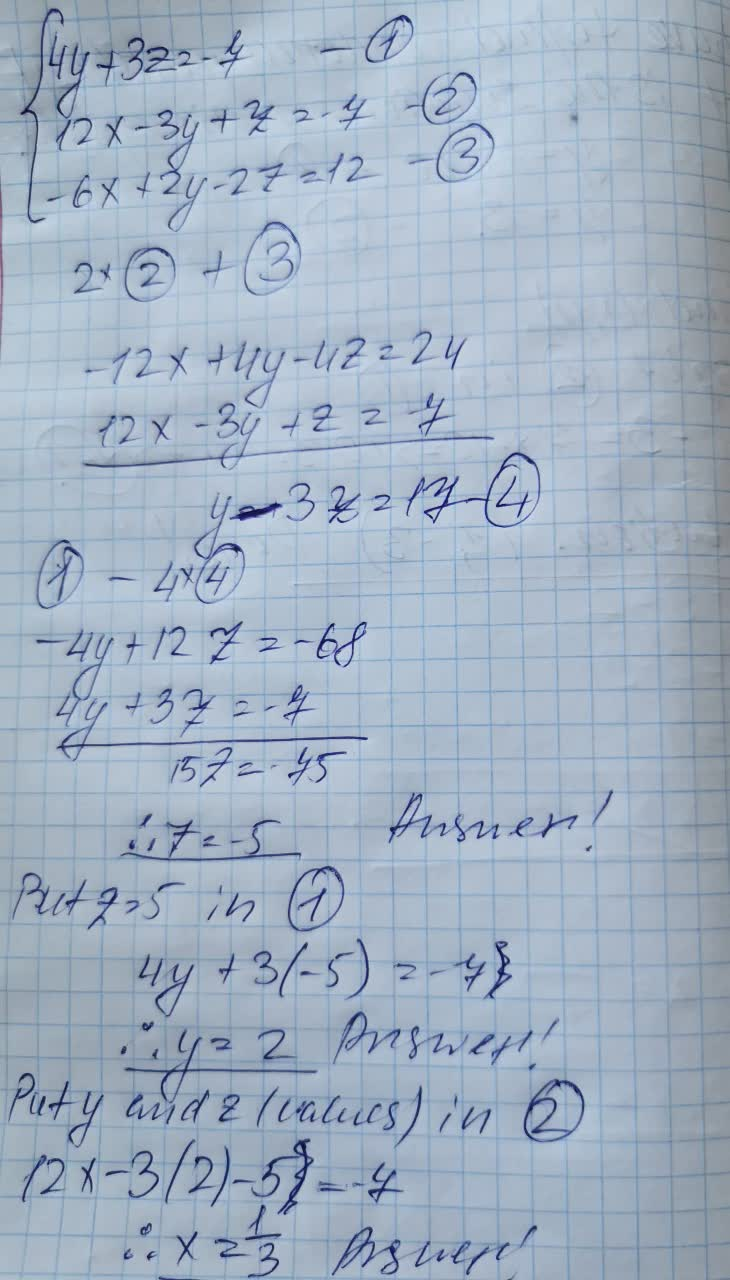# Solve the following systems of equations.4w+x4+2y-3z=-16, -3w+3x-ypt+4z=20, -w+2x+Sy+z=-4, 5w+4x+3y--z=-10Kaycee Roche 2021-09-09 Answered

Solve the following systems of equations.
$$\begin{cases}4y+3z=-7\\12x-3y+z=-7\\-6x+2y-27=12\end{cases}$$

• Questions are typically answered in as fast as 30 minutes

### Plainmath recommends

• Get a detailed answer even on the hardest topics.
• Ask an expert for a step-by-step guidance to learn to do it yourself.toroztatG

Solving this system of equations